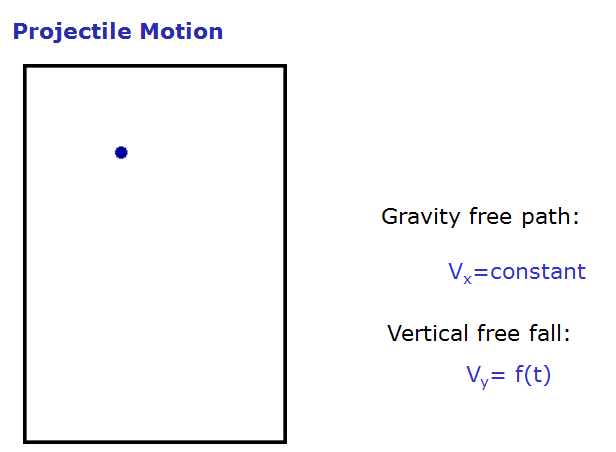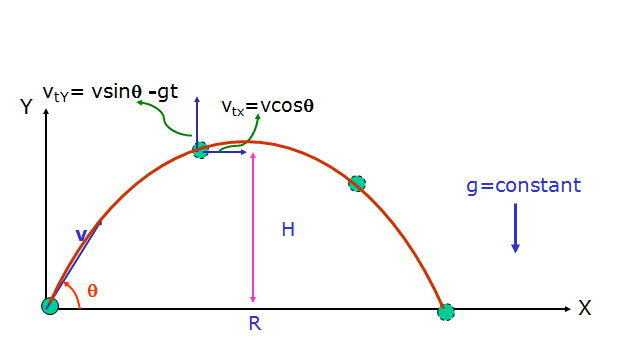SCC Education

Kinematics ,Projectile motion

Projectile motion (Definition)
Projectile Motion =
Uniform horizontal motion +
Non-Uniform vertical motion

(constant acceleration due to gravity)
Assumptions :
(1)  g=constant (y<<Rearth)
(2) v<<vescape
(3) Air friction & force of buoyancy are neglected
(4) Object is a particle    Scc-education.comOther Physics Topic.

Circular Motion ,centripetal force